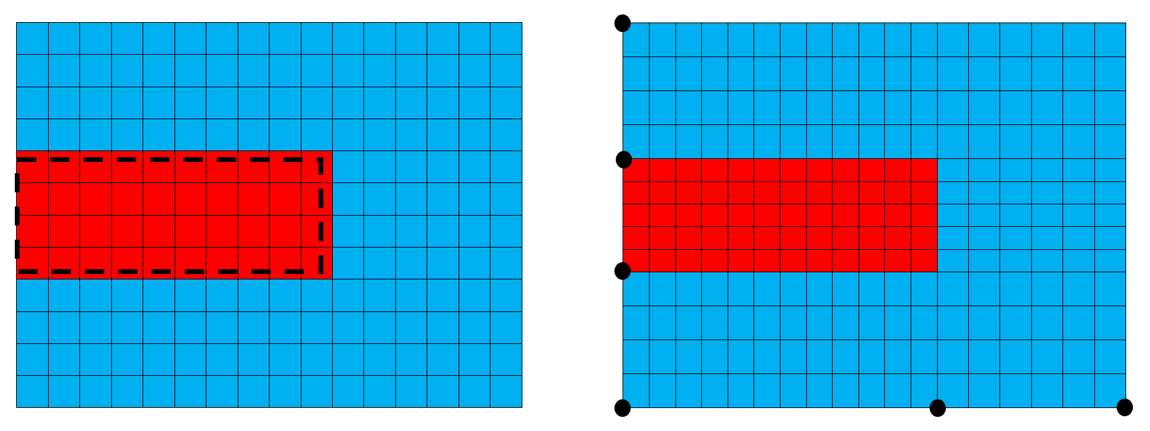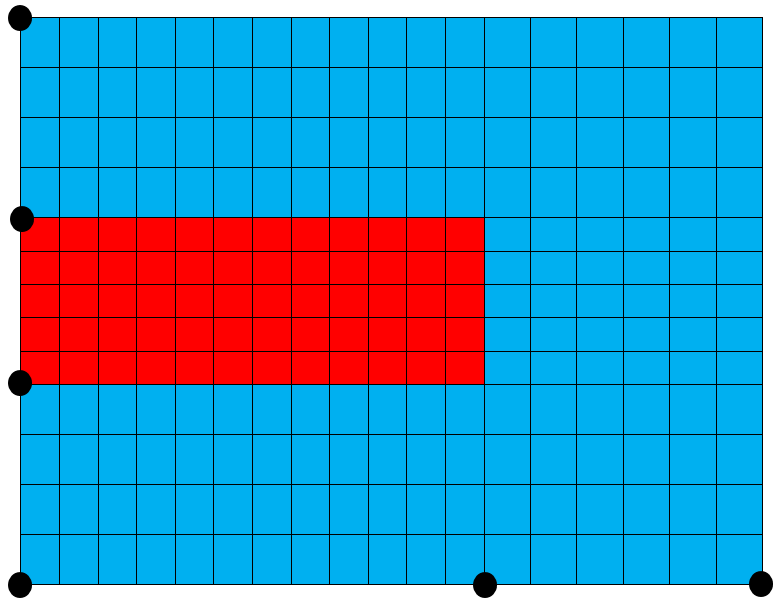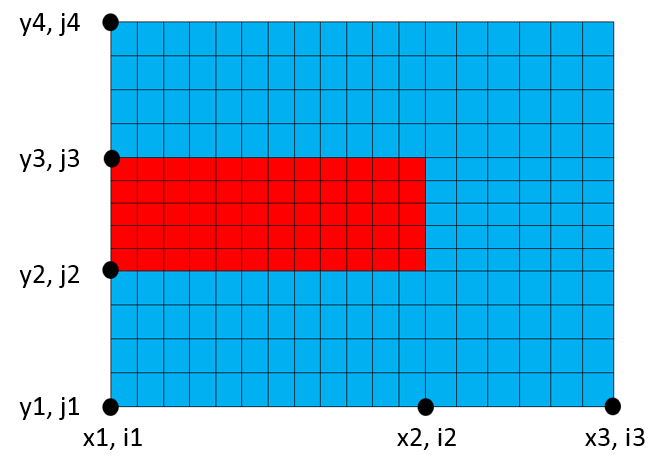# What are Keypoints?

When creating a model it is often beneficial to set up the grid using keypoints to allow for rapid parametrization and specific control of model. Keypoints are specific points in your model that identify:

• Important geometry considerations
• Definitive material boundaries
• Specific points for data extraction
• Specific regions for different meshing considerations

The keypoints define both xyz coordinates and ijk nodal locations of each point in a model:

• X dimension maps to I nodes
• Y dimension maps to J nodes
• Z dimension maps to K nodes

## Comparison with Standard Grid

The use of keypoints is not essential when creating a model and it is easy to create a regular spaced grid and impose the model geometry on to it. However, this can lead to slight errors in the model geometry dimensions. To help illustrate the benefit of using keypoints, the below images show a simple model geometry of a PZT disc in water and its grid when constructed using a regular spaced grid and when keypoints are used.Model geometry and grid when generated using (left) regular spaced grid and (right) keypoints

In the left image where a regular spaced grid is used, the actual size of the red PZT material is shown with a black dotted box. This highlights that the PZT geometry created is slightly incorrect due to the size of the grid elements. When we utilise keypoints, as shown by the black dots in the right image, it allows for nodes to be positioned exactly on the material boundary to ensure the model geometry is accurate. The element size between keypoints are either stretched or squeezed slightly to ensure the grid fits the keypoints.

## Generating Keypoints

Keypoints are generated by defining both incremental:

• XYZ values to represent their physical location in the model
• IJK integers to represent the nodal value at their location

This can be illustrated using the simple PZT disc example:PZT disc in water keypoint locations

In this example, the following commands can be used to define the keypoints:

```symb pzt_rad = 10.e-3          /* radius of pzt disc
symb pzt_thk = 4.e-3           /* thickness of pzt disc
symb water_size = 5.e-3        /* thickness of water layer around PZT

symb x1 = 0.                   /* x1 = 0.0 m, the origin
symb x2 = \$x1 + \$pzt_rad       /* x2 = 10.e-3 m
symb x3 = \$x2 + \$water_size    /* x3 = 15.e-3 m

symb y1 = 0.                   /* y1 = 0.0 m, the origin
symb y2 = \$y1 + \$water_size    /* y2 = 5.e-3 m
symb y3 = \$y2 + \$pzt_thk       /* y3 = 9.e-3 m
symb y4 = \$y3 + \$water_size    /* y4 = 14.e-3 m
```

To determine the nodal indices at each location we need to know the XYZ coordinates of the keypoints and the desired element size. We use this information to calculate the distance between keypoints and insert an integer number of elements between them to meet the required element size. This can be achieved using the following:

```symb i1 = 1                                          /* first node at origin
symb i2 = \$i1 + nint ( ( \$x2 - \$x1 )  / \$box )       /*calculate node at x2. nint rounds to nearest integer and box is the desired element size
symb i3 = \$i2 + nint ( ( \$x3 - \$x2 )  / \$box )
symb indgrd = \$i3                                    /*store the value of the last node number

symb j1 = 1                                          /* first node at origin
symb j2 = \$j1 + nint ( ( \$y2 - \$y1 )  / \$box )       /*calculate node at y2. nint rounds to nearest integer and box is the desired element size
symb j3 = \$j1 + nint ( ( \$y3 - \$y2 )  / \$box )
symb j4 = \$j3 + nint ( ( \$y4 - \$y3 )  / \$box )
symb jndgrd = \$j4                                    /*store the value of the last node number
```

## Generating the grid

The model grid can be generated using the GRID primary command:

`grid \$indgrd \$jndgrd`

The above line of code will create a 2D grid containing indgrd x jndgrd nodes.

## Linking XYZ Coordinates to IJK Indices

Once the grid has been generated, the XYZ location of the nodes needs to be set. This is done using the GEOM primary command:

```geom
xcrd \$x1 \$x2 \$i1 \$i2
xcrd \$x2 \$x3 \$i2 \$i3
ycrd \$y1 \$y2 \$j1 \$j2
ycrd \$y2 \$y3 \$j2 \$j3
ycrd \$y3 \$y4 \$j3 \$j4```

The above lines of code are identifying beginning and ending coordinates for a range of nodes in the grid. Taking the first sub-command will assign the node \$i1 at location \$x1 and node \$i2 at location \$x2, while the nodes in between will be linearly interpolated as shown below:Illustration of  mapping IJK nodes to XYZ coordinates using GEOM